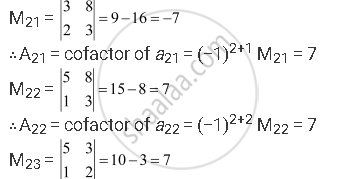Share

# Using Cofactors of Elements of Second Row, Evaluate Triangle = |(5,3,8),(2,0,1),(1,2, 3)| - Mathematics

#### Question

Using Cofactors of elements of second row, evaluate triangle = |(5,3,8),(2,0,1),(1,2, 3)|

#### Solution

The given determinant is  |(5,3,8),(2,0,1),(1,2, 3)|

We have:∴A23 = cofactor of a23 = (−1)2+3 M23 = −7

We know that Δ is equal to the sum of the product of the elements of the second row with their corresponding cofactors.

∴Δ = a21A21 + a22A22 + a23A23 = 2(7) + 0(7) + 1(−7) = 14 − 7 = 7

Is there an error in this question or solution?